### Problem Set 6 Trigonometry Class 10th Mathematics Part 2 MHB Solution

Problem Set 6
1. sinθ cosecθ = ? Choose the correct alternative answer for the following question.A. 1…
2. cosec45° = ? Choose the correct alternative answer for the following question.A.…
3. 1 + tan^2 θ = ? Choose the correct alternative answer for the following question.A.…
4. When we see at a higher level, from the horizontal line,angle formed is....... Choose…
5. If sintegrate heta = 11/61 find the values of cosθ using trigonometric identity.…
6. If tan θ = 2, find the values of other trigonometric ratios.
7. If sectheta = 13/12 find the values of other trigonometric ratios.…
8. secθ(1 - sinθ) (secθ + tanθ) = 1 Prove the following.
9. (secθ + tanθ) (1 - sinθ) = cosθ Prove the following.
10. sec^2 θ + cosec^2 θ = sec^2 θ × cosec^2 θ Prove the following.
11. cot^2 θ - tan^2 θ = cosec^2 θ - sec^2 θ Prove the following.
12. tan^4 θ + tan^2 θ = sec^4 θ - sec^2 θ Prove the following.
13. 1/1-sintegrate heta + 1/1+sintegrate heta = 2sec^2theta Prove the following.…
14. sec^6 x - tan^6 x = 1 + 3 sec^2 x × tan^2 x Prove the following.
15. tantheta /sectheta +1 = sectheta -1/tantheta Prove the following.…
16. tan^3theta -1/tantheta -1 = sec^2theta +tantheta Prove the following.…
17. sintegrate heta -costheta +1/sintegrate heta +costheta -1 = 1/sectheta -tantheta Prove…
18. A boy standing at a distance of 48 meters from a building observes the top of the…
19. From the top of the light house, an observer looks at a ship and finds the angle of…
20. Two buildings are in front of each other on a road of width 15 meters. From the top of…
21. A ladder on the platform of a fire brigade van can be elevated at an angle of 70° to…
22. While landing at an airport, a pilot made an angle of depression of 20°. Average speed…
###### Problem Set 6
Question 1.

Choose the correct alternative answer for the following question.

sinθ cosecθ = ?
A. 1

B. 0

C.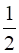D.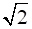We know,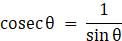⇒ sinθ cosecθ = 1

Question 2.

Choose the correct alternative answer for the following question.

cosec45° = ?
A.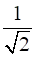B.C.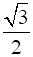D.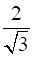As, cosec45° = √2

Question 3.

Choose the correct alternative answer for the following question.

1 + tan2θ = ?
A. cot2θ

B. cosec2θ

C. sec2θ

D. tan2θ

We know that,

1 + tan2θ = sec2θ

Question 4.

Choose the correct alternative answer for the following question.

When we see at a higher level, from the horizontal line,angle formed is.......
A. angle of elevation.

B. angle of depression.

C. 0

D. straight angle.

When we see at a higher level, from the horizontal line, the angle formed is known as angle of elevation.

Question 5.

If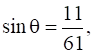find the values of cosθ using trigonometric identity.

As,

sin2θ + cos2θ = 1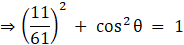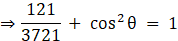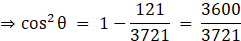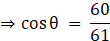Question 6.

If tan θ = 2, find the values of other trigonometric ratios.

We know that,

sec2θ= 1 + tan2θ

⇒ sec2θ = 1 + (2)2

⇒ sec2θ = 5

⇒ sec θ = √5 …

Also,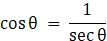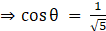…

Now, using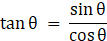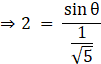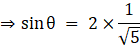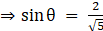…

Also,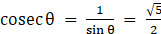…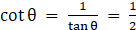….

Question 7.

If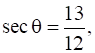find the values of other trigonometric ratios.

We know that,

sec2θ= 1 + tan2θ

⇒ tan2θ = sec2θ - 1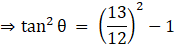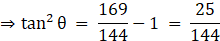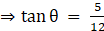… 

Also,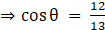… 

Now, using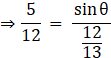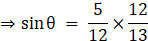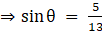… 

Also,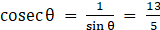…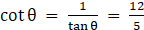….

Question 8.

Prove the following.

secθ(1 - sinθ) (secθ + tanθ) = 1

Taking LHS

secθ(1 - sinθ) (secθ + tanθ)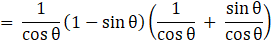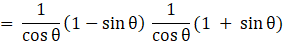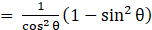[(a + b)(a - b) = a2 - b2]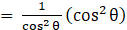[ sin2θ + cos2θ = 1]

= 1

= RHS

Proved !

Question 9.

Prove the following.

(secθ + tanθ) (1 - sinθ) = cosθ

Taking LHS

(1 - sinθ) (secθ + tanθ)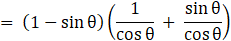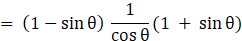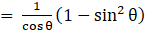[(a + b)(a - b) = a2 - b2]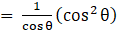[ sin2θ + cos2θ = 1]

= cos θ

= RHS

Proved !

Question 10.

Prove the following.

sec2θ + cosec2θ = sec2θ × cosec2θ

Taking LHS

Sec2θ + cosec2θ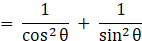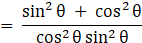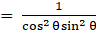[ sin2θ + cos2θ = 1]

= sec2θ × cosec2θ

= RHS

Proved !

Question 11.

Prove the following.

cot2θ - tan2θ = cosec2θ - sec2θ

Taking LHS

cot2θ - tan2θ

[ Now, cosec2θ - 1 = cot2θ and sec2θ - 1 = tan2θ]

= cosec2θ - 1 - (sec2θ - 1)

= cosec2θ - sec2θ

= RHS

Proved !

Question 12.

Prove the following.

tan4θ + tan2θ = sec4θ - sec2θ

Taking LHS

tan4θ + tan2θ

= tan2θ( tan2θ + 1)

= (sec2θ - 1)(sec2θ) [1 + tan2θ = sec2θ]

= sec4θ - sec2θ

= RHS

Proved !

Question 13.

Prove the following.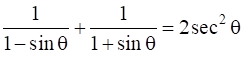Taking LHS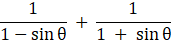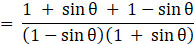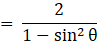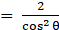[ sin2θ + cos2θ = 1]

= 2 sec2θ

= RHS

= Proved

Question 14.

Prove the following.

sec6x – tan6x = 1 + 3 sec2x × tan2x

Taking LHS

sec6x - tan6x

= (sec2x)3 - (tan2x)3

= (sec2x - tan2x)(sec4x + tan2x sec2x + tan4x)

[As, a3 - b3 = (a - b)(a2 + ab + b2)]

= sec4x + tan4x + tan2x sec2x + 2tan2x sec2x - 2tan2x sec2x

[As, sec2θ - tan2θ = 1]

= sec4x + tan4x - 2tan2x sec2x + 3tan2x sec2x

= (sec2x - tan2x)2 + 3tan2x sec2x [a2 + b2 - 2ab = (a - b)2]

= 12 + 3tan2x sec2x

= 1 + 3tan2x sec2x

= RHS

Proved.

Question 15.

Prove the following.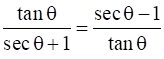Taking LHS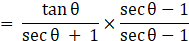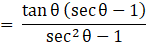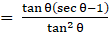[tan2θ = sec2θ - 1]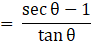= RHS

Proved !

Question 16.

Prove the following.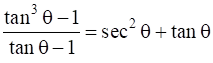Taking LHS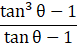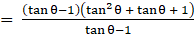[a3 - b3 = (a - b)(a2 + ab + b2)]

= tan2θ + tanθ + 1

= sec2θ + tanθ [1 + tan2θ = sec2θ]

= RHS
Proved.

Question 17.

Prove the following.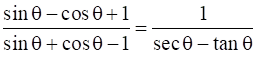Taking LHS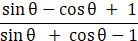Dividing numerator and denominator by cosθ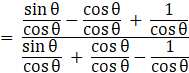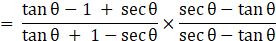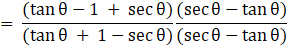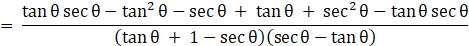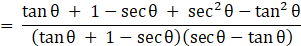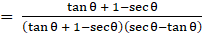[As sec2θ - tan2θ = 1]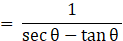= RHS

Proved.

Question 18.

A boy standing at a distance of 48 meters from a building observes the top of the building and makes an angle of elevation of 30°. Find the height of the building.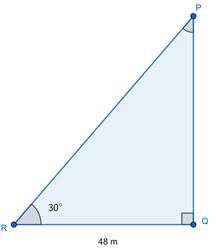Let 'R' be the person, standing 48 m away from a building PQ,

Angle of elevation, ∠PRQ = θ = 30°

Clearly, ∆ABC is a right-angled triangle, in which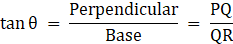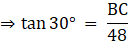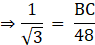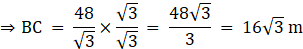Therefore, Height of church is 16√3 m.

Question 19.

From the top of the light house, an observer looks at a ship and finds the angle of depression to be 30°. If the height of the light-house is 100 meters, then find how far the ship is from the light-house.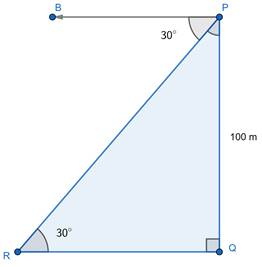Let PQ be a light house of height 80 cm such that PQ = 100 m

And R be a ship.

Angle of depression from P to ship R = ∠BPR = 30°

Also, ∠PRQ(say θ) = ∠BPR = 30° [Alternate Angles]

Clearly, PQR is a right-angled triangle.

Now, In ∆PQR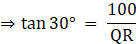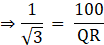⇒ QR = 100√3 m

Hence, Ship is 100√3 m away from the light house.

Question 20.

Two buildings are in front of each other on a road of width 15 meters. From the top of the first building, having a height of 12 meter, the angle of elevation of the top of the second building is 30°.What is the height of the second building?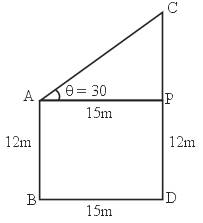Let AB and CD be two building, with

AB = 12 m

And angle of elevation from top of AB to top of CD = ∠CAP = 30°

Width of road = BD = 15 m

Clearly, ABDP is a rectangle

With

AB = PD = 12 m

BD = AP = 15 m

And APC is a right-angled triangle, In ∆APC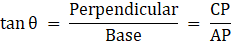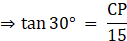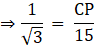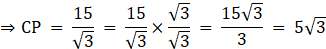⇒ CP = 5√3 m

Also,

CD = CP + PD = (5√3 + 12) m

Hence, height of other building is (12 + 5√3 m).

Question 21.

A ladder on the platform of a fire brigade van can be elevated at an angle of 70° to the maximum. The length of the ladder can be extended upto 20m. If the platform is 2m above the ground, find the maximum height from the ground upto which the ladder can reach. (sin 70° = 0.94)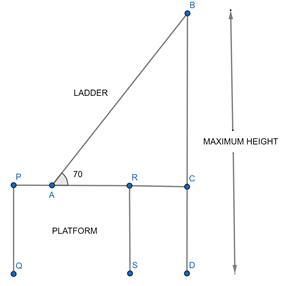Let AB be the ladder, i.e. AB = 20 m and PQRS be the platform.

In the above figure, clearly

PQ = RS = CD = height of platform = 2 m

Maximum height ladder can reach = BC + CD

Now,

Maximum value of ∠BAC = 70°

In right-angled triangle ABC,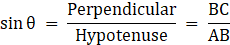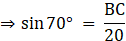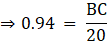⇒ BC = 18.8 m

Maximum height ladder can reach = BC + CD

= 18.8 + 2 = 20.8 meters

Question 22.

While landing at an airport, a pilot made an angle of depression of 20°. Average speed of the plane was 200 km/hr. The plane reached the ground after 54 seconds. Find the height at which the plane was when it started landing. (sin 20° = 0.342)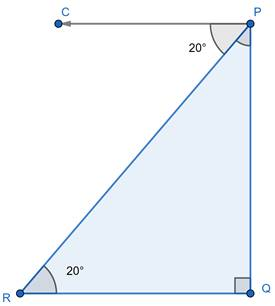Let the initial position of plain be P and after landing it position be R.

Now,

Angle of depression while landing, ∠CPR = 20°

Also, ∠ CPR = ∠PRQ (say θ) = 20° [Alternate Angles]

Now,

Speed of plane = 200 km / hr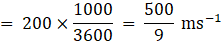Time taken for landing = 54 seconds

Distance travelled in landing = PR

Also, distance = speed × time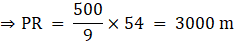Now, In ∆PQR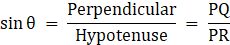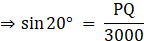⇒ PQ = 3000 × sin 20°

⇒ PQ = 3000(0.342)

⇒ PQ = 1026 m

So, Plane was at a height of 1026 m at the start of landing.

## PDF FILE TO YOUR EMAIL IMMEDIATELY PURCHASE NOTES & PAPER SOLUTION. @ Rs. 50/- each (GST extra)

SUBJECTS

HINDI ENTIRE PAPER SOLUTION

MARATHI PAPER SOLUTION
SSC MATHS I PAPER SOLUTION
SSC MATHS II PAPER SOLUTION
SSC SCIENCE I PAPER SOLUTION
SSC SCIENCE II PAPER SOLUTION
SSC ENGLISH PAPER SOLUTION
SSC & HSC ENGLISH WRITING SKILL
HSC ACCOUNTS NOTES
HSC OCM NOTES
HSC ECONOMICS NOTES
HSC SECRETARIAL PRACTICE NOTES

2019 Board Paper Solution

HSC ENGLISH SET A 2019 21st February, 2019

HSC ENGLISH SET B 2019 21st February, 2019

HSC ENGLISH SET C 2019 21st February, 2019

HSC ENGLISH SET D 2019 21st February, 2019

SECRETARIAL PRACTICE (S.P) 2019 25th February, 2019

HSC XII PHYSICS 2019 25th February, 2019

CHEMISTRY XII HSC SOLUTION 27th, February, 2019

OCM PAPER SOLUTION 2019 27th, February, 2019

HSC MATHS PAPER SOLUTION COMMERCE, 2nd March, 2019

HSC MATHS PAPER SOLUTION SCIENCE 2nd, March, 2019

SSC ENGLISH STD 10 5TH MARCH, 2019.

HSC XII ACCOUNTS 2019 6th March, 2019

HSC XII BIOLOGY 2019 6TH March, 2019

HSC XII ECONOMICS 9Th March 2019

SSC Maths I March 2019 Solution 10th Standard11th, March, 2019

SSC MATHS II MARCH 2019 SOLUTION 10TH STD.13th March, 2019

SSC SCIENCE I MARCH 2019 SOLUTION 10TH STD. 15th March, 2019.

SSC SCIENCE II MARCH 2019 SOLUTION 10TH STD. 18th March, 2019.

SSC SOCIAL SCIENCE I MARCH 2019 SOLUTION20th March, 2019

SSC SOCIAL SCIENCE II MARCH 2019 SOLUTION, 22nd March, 2019

XII CBSE - BOARD - MARCH - 2019 ENGLISH - QP + SOLUTIONS, 2nd March, 2019

HSC Maharashtra Board Papers 2020

(Std 12th English Medium)

HSC ECONOMICS MARCH 2020

HSC OCM MARCH 2020

HSC ACCOUNTS MARCH 2020

HSC S.P. MARCH 2020

HSC ENGLISH MARCH 2020

HSC HINDI MARCH 2020

HSC MARATHI MARCH 2020

HSC MATHS MARCH 2020

SSC Maharashtra Board Papers 2020

(Std 10th English Medium)

English MARCH 2020

HindI MARCH 2020

Hindi (Composite) MARCH 2020

Marathi MARCH 2020

Mathematics (Paper 1) MARCH 2020

Mathematics (Paper 2) MARCH 2020

Sanskrit MARCH 2020

Important-formula

THANKS